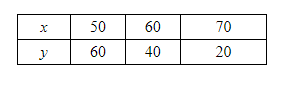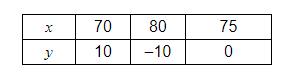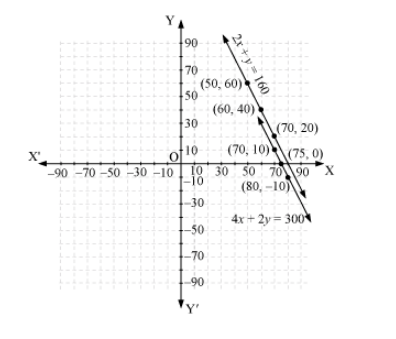# The cost of 2kg of apples and 1 kg of grapes on a day was found to be Rs 160.`
Question:

The cost of 2kg of apples and 1 kg of grapes on a day was found to be Rs 160. After a month, the cost of 4 kg of apples and 2kg of grapes is Rs. 300 Represent th situation algebraically and geometrically.

Solution:

Let the cost of 1 kg of apples be Rs x.

And, cost of 1 kg of grapes = Rs y

According to the question, the algebraic representation is

$2 x+y=160$

$4 x+2 y=300$

For $2 x+y=160$,

$y=160-2 x$

The solution table isFor $4 x+2 y=300$

$y=\frac{300-4 x}{2}$

The solution table isThe graphical representation is as follows.